# Python Dictionary | Create, Add, Get, and Delete Dictionary in Python

Dictionary in Python is a data type, a kind of mapping type, that is used when we need to store multiple items in the form of key-value pairs.

In the real world, you can consider any dictionary book where the information is stored in the form of a key-value pair, where the key refers to the word and the value refers to its definition.

The dictionary helps organize the information. For example, in a dictionary book, all the words are organized in alphabetical order so that we can easily find the required word and get the definition or description of that word very easily.

Note: A dictionary in Python is an ordered and changeable (mutable) collection of items where duplicates aren't allowed.

### What are the topics covered in this post?

This post deals with the following topics regarding the "dictionary" in Python:

## Create a dictionary in Python

In Python, a dictionary is created in key-value pairs, where there is a colon (:) in between key and value and a comma (,) in between every item. Each item contains a key and its value. Also, the whole item must be enclosed within a curly brace, i.e. {}, Here is the syntax to create a dictionary in Python:

`mydict = {key_1: val_1, key_2: val_2, ..., key_n: val_n}`

Here key_1, key_2, and key_n are keys, whereas val_1, val_2, and val_n are values. Here is a simple example of a dictionary:

```mydict = {"Day": "Wednesday", "Month": "October"}
print(mydict)```

The output produced by the above Python program on the dictionary is shown in the snapshot given below: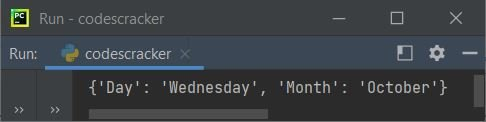You're seeing the whole dictionary in the output. To access and print a specific value of a specific key, use mydict[key]. You'll learn all about accessing keys, values, or items of a dictionary later on in this article.

Important: While creating a dictionary, always keep in mind that keys of the dictionary must be a single element, whereas values may be a single element such as an integer, string, etc., or a multiple element such as a list, tuple, etc.

Note: When creating a dictionary with each item in pairs, the colon (:) is not necessary between the key and value. A demonstration of it will be provided later in this tutorial.

### Make a dictionary with several keys

The data type of all keys in a single dictionary may be the same or different. Here is an example that uses multiple types of keys:

`my_dictionary = {1: "Python", "city": "New York City"}`

As you can see, the first key, 1, is of integer type, whereas the second key, "city," is of string type.

### Create a dictionary with multiple values for a single key

As already mentioned in the Important Notice,  the value of a single key in a dictionary may be a single or multiple values. Here is an example: assigning multiple values to a key named Marks.

`my_dictionary = {"Name": "Luis", "Marks": [89, 96, 73]}`

In the above statement, [89, 96, 73] is basically a list of three elements (numbers), and this list is the value of a key named Marks.

### Create an empty dictionary

To create an empty dictionary, don't add any keys or values inside the curly braces. Here is an example of how to create an empty dictionary in Python:

`my_dictionary = {}`

An empty dictionary can also be created using the dict() method, as shown below:

`my_dictionary = dict()`

### Create a dictionary using the dict() method

A dictionary can also be created using the dict() method. Here is an example:

```my_dictionary = dict({"Name": "Jonah", "Marks": [89, 93, 97]})
print(my_dictionary)```

The output produced by the above program will be:

`{'Name': 'Jonah', 'Marks': [89, 93, 97]}`

### Create a dictionary with each item in pairs

While creating a dictionary in which each item is in pairs, we do not need to use a colon (:) to separate key and value. Also, there is no need for curly braces. Here is an example that shows how to create a dictionary with each item in pairs.

```my_dictionary = dict([("Name", "Jonah"), ("Marks", [89, 93, 97])])
print(my_dictionary)```

The output of this program will be identical to that of the previous program.

### Create a nested dictionary

A dictionary can also be nested inside another. Here is an example of a nested dictionary in Python:

```my_dictionary = {"College": "Harvard University", "Program": "Computer Science",
"Student": {"Name": "Nathaniel", "Address": "1549 Scott Center Rd Sherman, New York(NY)",
"Marks": [97, 98, 85]}}
print(my_dictionary)
```

The output produced by the above Python program on creating a nested dictionary is:

`{'College': 'Harvard University', 'Program': 'Computer Science', 'Student': {'Name': 'Nathaniel', 'Address': '1549 Scott Center Rd Sherman, New York(NY)', 'Marks': [97, 98, 85]}}`

In the above program, the values of the key named "Student" are themselves a dictionary, in which there are three items.

## Get dictionary keys, values, or items in Python

This section is an important one; it shows how to get or access only keys, only values, or the whole contents of a dictionary. Let's start with accessing the values of a dictionary using its key.

### Get a value from a dictionary using its key

A dictionary value can be accessed using its key. Here is the syntax:

`my_dictionary[key];`

where "key" is the name of the key whose value is going to be accessed. For example:

```mydict = {"Day": "Wednesday", "Month": "October"}
print(mydict["Day"])
print(mydict["Month"])```

The snapshot given below shows the sample output produced by the above program:The question is, what if the key whose value is going to be accessed is not available in the dictionary?
Let's find out using the program given below that accesses a key from the dictionary that is not available:

```mydict = {"Name": "Jonah", "City": "Tacoma"}
print(mydict["Marks"])```

Since the key named "Marks" is not available in the dictionary named "mydict," the above program produced or raised an error, as shown in the snapshot given below: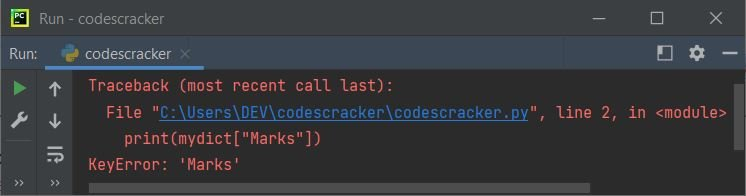Since the error raised is a KeyError, to handle this error, we need to use a try-except block as shown in the example given below:

```mydict = {"Name": "Jonah", "City": "Tacoma"}
try:
print(mydict["Marks"])
except KeyError:
print("\nThe key named \"Marks\" is not available!")```

Now the output will be:

`The key named "Marks" is not available!`

The get() method can also be used to access a dictionary's values using keys, as shown in the example given below:

```mydict = {"Day": "Wednesday", "Month": "October"}
print(mydict.get("Day"))
print(mydict.get("Month"))```

The output will be the same as the previous program's output.

Note: Using the get() method to access an unavailable key of a dictionary, for example, "print(mydict.get("Marks"))" produces "None."

### Get nested dictionary values

To get nested dictionary values in Python, we need to nest keys. Here is the syntax to get values available in a nested dictionary:

`my_dictionary[key_one][key_two]`

The above code returns the value of the key named "key_two." The "key_two" is one of the keys available inside a nested dictionary, which is the value of a key named "key_one." Here is an example of accessing values available in a nested dictionary:

```my_dictionary = {"College": "Harvard University", "Program": "Computer Science",
"Student": {"Name": "Nathaniel", "Address": "1549 Scott Center Rd Sherman, New York(NY)",
"Marks": [97, 98, 85]}}

The above program displays the values of keys named Address and Student. Here is the output:

`1549 Scott Center Rd Sherman, New York(NY)`

### Print the Dictionary's Key

Sometimes we need to see the names of all the keys available in a dictionary. As a result, here is the code I wrote to list and print all keys in a dictionary called mydict:

```mydict = {"Name": "Jonah", "City": "Forks"}
for d in mydict:
print(d)```

Here is its sample output:

```Name
City```

### Print All Values in the Dictionary

Most of the time, we need to see all values of the dictionary, except the whole dictionary. Therefore, here is the program I've created that shows how to access and print all values of a dictionary in Python:

```mydict = {"Name": "Jonah", "City": "Forks"}
for i in mydict:
print(mydict[i])```

Here is its sample output:

```Jonah
Forks```

The above program can also be replaced with the following program. This program uses the values() method to do the same task of printing all dictionary values:

```mydict = {"Name": "Jonah", "City": "Forks"}
for i in mydict.values():
print(i)```

This program produces exactly the same output as the previous one.

### Print Keys and Values of the Dictionary

This is the program I think everyone needs to get while learning a dictionary in Python. Because getting and printing all the keys and values of a dictionary in a manual way is needed most of the time. Therefore, I've created a program that shows how to print keys and values of a dictionary without its default format using manually formatted output:

```mydict = {"Name": "Jonah", "City": "Tacoma"}

print("Key\t\t\t Value")
print("----------------------")
for i in mydict:
print(i, "\t\t", mydict[i])```

The output produced by the above program will be: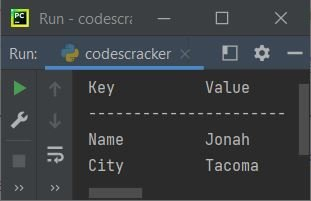### Print the entire dictionary

This program prints all items of a dictionary using the items() method. Let's take a look at the program given below:

```mydict = {"Name": "Mark", "Program": "Computer Science", "Age": 22}
for i in mydict.items():
print(i)```

The output produced by the above program will be:

```('Name', 'Mark')
('Program', 'Computer Science')
('Age', 22)```

The first element of each item indicates the key, whereas the second element of each item indicates the value.

Also, we can print both keys and values of the dictionary using the same technique as in the above program. Here is the program that prints both keys and values of the dictionary in manual format:

```mydict = {"Name": "Mark", "Program": "Computer Science", "Age": 22}
for i, j in mydict.items():
print(i, "->", j)```

This Python program's output will be the following:## Add a New Item to a Dictionary in Python

To add a new item to a dictionary, here is the syntax:

`my_dictionary[key] = value`

Or use the update() method to add a new item to the dictionary. Another syntax that uses the update() method to accomplish the same task as above:

`my_dictionary.update({key: value})`

Note: For key and/or value of string type, use double quotes ("") to enclose the key and/or value.

Important: When you add a new item to the dictionary using either of the two methods described above, the item will be added at the end of the dictionary. And if the name of the key is already available in the dictionary, then the value of that key will get updated. It is because the dictionary does not allow duplicates.

Here is an example of adding or updating an item to the dictionary based on user input:

```print("Enter 2 Items for the Dictionary.")
my_dictionary = {}
for i in range(2):
print("Enter Key No.", i+1, ": ", end="", sep="")
key = input()
print("Enter Value for Key No.", i+1, ": ", end="", sep="")
value = input()
my_dictionary.update({key: value})

print("\nThe Dictionary is:")
print(my_dictionary)```

Here is its sample run with user inputs, with "First Key" and "First Value" as key and value for the first item, and "Second Key" and "Second Value" as key and value for the second item of the dictionary: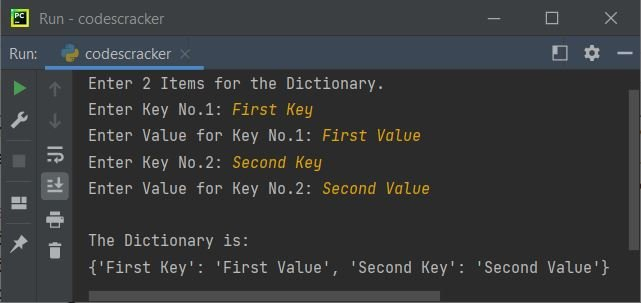Note: The end and sep are the two parameters of print() that are used to change the default behavior of the print statement.

Note: Since by default, the input() method treats every entered value as a string type, That is, whatever the value you enter, it gets converted into a string automatically.

Now the example given below shows how the value gets updated if the key used to add a new item already exists in the dictionary:

```mydict = {"Name": "Jonah", "City": "Seattle"}
print(mydict)
mydict["City"] = "Bellingham"
print(mydict)```

The output produced by the above program will be:

```{'Name': 'Jonah', 'City': 'Seattle'}
{'Name': 'Jonah', 'City': 'Bellingham'}```

## In Python, find the length of a dictionary

To find the length of a dictionary, use the len() method as shown in the program given below:

```my_dict = {1: "USA", 2: "UK", 3: "Australia"}
my_dict_len = len(my_dict)
print("The dictionary \"my_dict\" has", my_dict_len, "items.")```

This will produce the following output:

`The dictionary "my_dict" has 3 items.`

## Delete an item from a dictionary in Python

To delete an item from a dictionary, use the del keyword as shown in the example given below:

```mydict = {"Day": "Wednesday", "Month": "October"}
print(mydict)

del mydict["Day"]
print(mydict)```

The output produced by the above Python program will exactly be: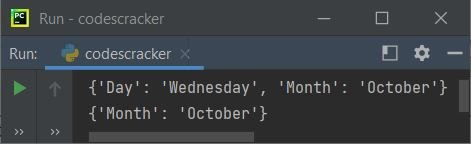As you can see from the above output, the second print of the dictionary has the first item, whose key's name is "Day," deleted.

The pop() method can also be used to pop or remove an item from the dictionary using its key name, as shown in the example given below:

```mydict = {"Day": "Wednesday", "Month": "October"}
print(mydict)

mydict.pop("Day")
print(mydict)```

The output will be identical to the previous program's.

### Delete the last item from a dictionary

To delete the last item from a dictionary, use popitem() as shown in the example given below:

```mydict = {"Day": "Wednesday", "Month": "October"}
print(mydict)

mydict.popitem()
print(mydict)

mydict.popitem()
print(mydict)```

The snapshot given below shows the sample output produced by the above Python program: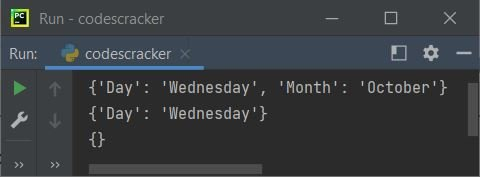Note: Every time we use popitem(), the last item will get deleted from the dictionary.

Now the question is, what if the dictionary is or becomes empty and we use popitem()?
Let's check it out using the program given below:

```mydict = {"Name": "Jonah", "City": "Olympia"}
print(mydict)

mydict.popitem()
print(mydict)

mydict.popitem()
print(mydict)

mydict.popitem()
print(mydict)```

The output this time will be: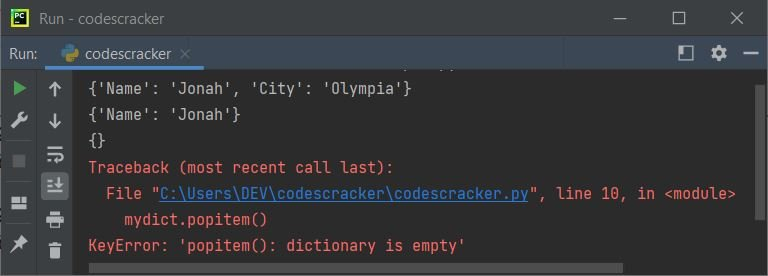See the error KeyError: 'popitem(): dictionary is empty' saying that the dictionary is empty, therefore no key is available to pop out. So we need to handle that KeyError error raised by using popitem() further when no item is available in the dictionary, as shown in the example given below:

```mydict = {"Name": "Jonah", "City": "Yakima"}
print(mydict)

try:
mydict.popitem()
print(mydict)
except KeyError:
print("\nThe Dictionary \"mydict\" is empty!")

try:
mydict.popitem()
print(mydict)
except KeyError:
print("\nThe Dictionary \"mydict\" is empty!")

try:
mydict.popitem()
print(mydict)
except KeyError:
print("\nThe Dictionary \"mydict\" is empty!")```

Since I want to make the program more dynamic, I assume that I don't know that at popitem(), the dictionary is empty. Therefore, I've added a try-except block to every  popitem() to catch the error when it's raised. Here is the output produced by the above program, this time: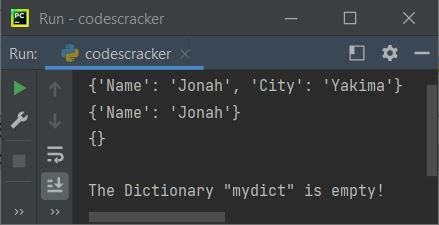Since the same statement is written three times, it is a better practice to wrap all those statements, written multiple times with exactly the same code, in a function. Here is the modified version of the previous program:

```def p(d):
try:
d.popitem()
print(mydict)
except KeyError:
print("\nThe Dictionary \"mydict\" is empty!")

mydict = {"Name": "Jonah", "City": "Yakima"}
print(mydict)

p(mydict)
p(mydict)
p(mydict)```

The output will be identical to that of the previous program.

### Delete All Dictionary Items at Once

To delete all items from a dictionary at once, use the clear() method as shown in the example given below:

```mydict = {"Name": "Jonah", "City": "Everett"}
print(mydict)

mydict.clear()
print(mydict)```

The output produced by the above program will exactly be:

```{'Name': 'Jonah', 'City': 'Everett'}
{}```

see the output. That is, before using the clear() method, we're seeing all the items in the dictionary. But after using the clear() method, we're not seeing any items because all the items are cleared.

### Delete the whole dictionary

The "del" keyword can also be used to delete the whole dictionary, as shown in the example given below.

```mydict = {"Day": "Wednesday", "Month": "October"}
print(mydict)

del mydict
print(mydict)```

The output of the preceding program will now produce the dictionary using first print() and an error using second print(). Because the dictionary is deleted using the del keyword before the second print() statement, Let's see the output first, and then we will see how to handle the error: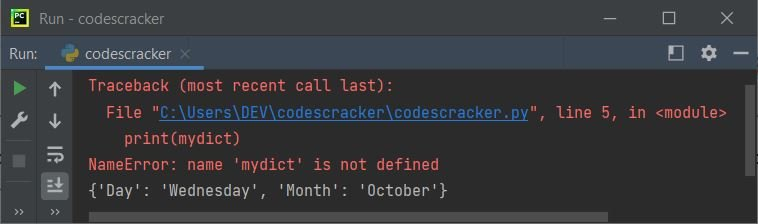since the name of the raised error is "NameError." Therefore, we need to handle this exception. Here is the demo program that shows how to handle these types of errors:

```mydict = {"Day": "Wednesday", "Month": "October"}
print(mydict)

del mydict
try:
print(mydict)
except NameError:
print("\nThe dictionary \"mydict\" is empty!")```

Now the output produced by the above program will be: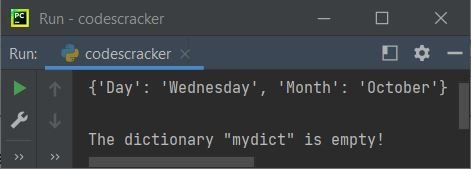Since we already know that the second print() raises an error while printing the dictionary mydict, I've applied the try-except block to handle the error raised by the second  print(). But you can put everything where you can check whether the dictionary we're going to print is empty or has some items.

## Dictionary Comprehension in Python

We can also use iterables to create a dictionary in a concise way, using small codes or single statements.

```cubes = {n: n*n*n for n in range(1, 6)}
print(cubes)```

In the above program, before : (the colon) refers to keys, whereas after : refers to values. since the range() method returns a sequence of values. Therefore, range (1, 6)  returns 1, 2, 3, 4, and 5. So the above program can also be written as:

```cubes = {n: n*n*n for n in (1, 2, 3, 4, 5)}
print(cubes)```

The output of this and the previous program is identical,

`{1: 1, 2: 8, 3: 27, 4: 64, 5: 125}`

The value of n will be 1 at first. Therefore, n: n*n*n or 1: 1*1*1 or 1: 1 will be initialized to cubes as its first item. Similarly n: n*n*n or 2: 2*2*2 or 2: 8 will get initialized to cubes as its second item, and so on.

The above and previous program can also be written as:

```cubes = {}
for i in range(1, 6):
cubes[i] = i*i*i
print(cubes)```

Here is another example: printing squares or cubes of the first 5 natural numbers based on user inputs:

```print("1. Squares")
print("2. Cubes")
print("Enter Your Choice (1 or 2): ", end="")
try:
ch_1 = int(input())
if ch_1==1:
print("\n1. Squares of First 5 Even Natural Numbers")
print("2. Squares of First 5 Odd Natural Numbers")
print("Enter Your Choice (1 or 2): ", end="")
try:
ch_2 = int(input())
if ch_2==1:
squares = {n: n*n for n in range(1, 11) if n%2==0}
print("\n", squares, sep="")
elif ch_2==2:
squares = {n: n*n for n in range(1, 11) if n%2!=0}
print("\n", squares, sep="")
except ValueError:
print("\nInvalid Input!")
elif ch_1==2:
print("\n1. Cubes of First 5 Even Natural Numbers")
print("2. Cubes of First 5 Odd Natural Numbers")
print("Enter Your Choice (1 or 2): ", end="")
try:
ch_2 = int(input())
if ch_2==1:
cubes = {n: n*n*n for n in range(1, 11) if n%2==0}
print("\n", cubes, sep="")
elif ch_2==2:
cubes = {n: n*n*n for n in range(1, 11) if n%2!=0}
print("\n", cubes, sep="")
except ValueError:
print("\nInvalid Input!")
except ValueError:
print("\nInvalid Input!")```

Here is its sample run with user inputs 1 and 2 as two choices: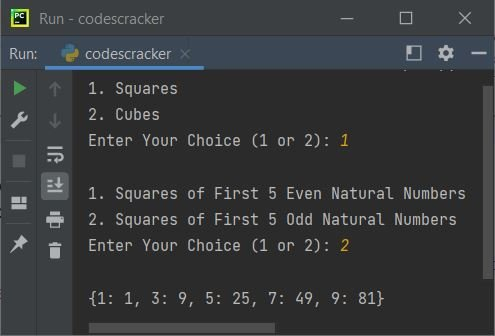This program is created to show you how we can apply the condition while creating a dictionary using dictionary comprehension.

## Mega Program on the Dictionary in Python

This is the final and most comprehensive dictionary program in Python. Concentrate on this program to learn a few extra things in Python:

```mydict = {}
while True:
print("\n1. Create Dictionary")
print("2. Show Dictionary")
print("4. Update Dictionary")
print("5. Delete Specific Item from Dictionary")
print("6. Delete All Items from Dictionary")
print("7. Exit")
print("Enter Your Choice (1-7): ", end="")
try:
ch = int(input())
if ch == 1:
print("\nHow many items to store in dictionary ? ", end="")
try:
tot = int(input())
print("Enter", tot, "Items for the dictionary.")
for i in range(tot):
print("\nEnter key and value for item no.", i+1, ": ", end="", sep="")
key = input()
value = input()
mydict[key] = value
except ValueError:
print("\nInvalid Input!")
elif ch == 2:
print("\n----The Dictionary is----")
for k, v in mydict.items():
print(k, "->", v)
elif ch == 3:
print("\nHow many items to add ? ", end="")
try:
tot = int(input())
print("Enter", tot, "Items for the dictionary.")
for i in range(tot):
print("\nEnter key and value for item no.", i+1, ": ", end="", sep="")
key = input()
if key in mydict:
print("The key \"", key, "\" is already available!", sep="")
else:
value = input()
mydict[key] = value
except ValueError:
print("\nInvalid Input!")
elif ch == 4:
print("\nHow many item to update ? ", end="")
try:
tot = int(input())
if tot > len(mydict):
print("\nThere is only", len(mydict), "items in the dictionary!")
else:
for i in range(tot):
print("Which key to update ? ", end="")
key = input()
if key in mydict:
print("Enter new value for this key: ", end="")
value = input()
mydict[key] = value
else:
except ValueError:
print("\nInvalid Input!")
elif ch == 5:
print("\nHow many item to delete ? ", end="")
try:
tot = int(input())
if tot > len(mydict):
print("\nThere is only", len(mydict), "items in the dictionary!")
else:
for i in range(tot):
print("Which key to delete ? ", end="")
key = input()
if key in mydict:
del mydict[key]
else:
except ValueError:
print("\nInvalid Input!")
elif ch == 6:
print("\nAre you sure to delete all items ? (y/n): ", end="")
c = input()
if c=='y':
mydict.clear()
print("The dictionary is now empty!")
elif ch == 7:
break
else:
print("\nInvalid Choice!")
except ValueError:
print("\nInvalid Choice!")```

Here is its sample run with user input 1 as a choice and 2 as the number of items to insert or add, then "First Key" and "First Value" as the first item, whereas "Second Key" and "Second Value" as the second item to add: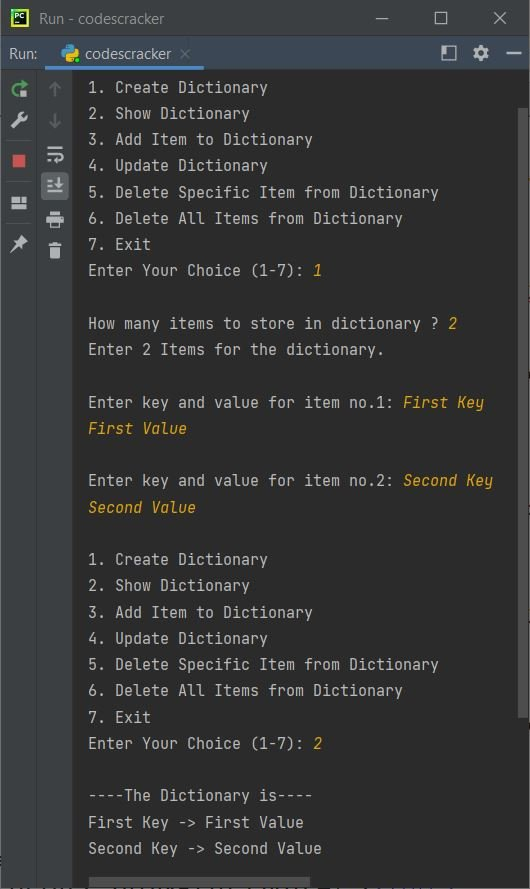Because the entire program is wrapped in a "while" loop with a condition of True, it always evaluates to True until a "break" keyword occurs, which occurs when you enter "7" as a choice to exit the program. Therefore, after displaying the output, the program still continues its execution. So let's make another choice. This time, I'm going with 4 as the choice to update, then 1 as the number of items to update, First Key as the key, and Value of First Key as its new value: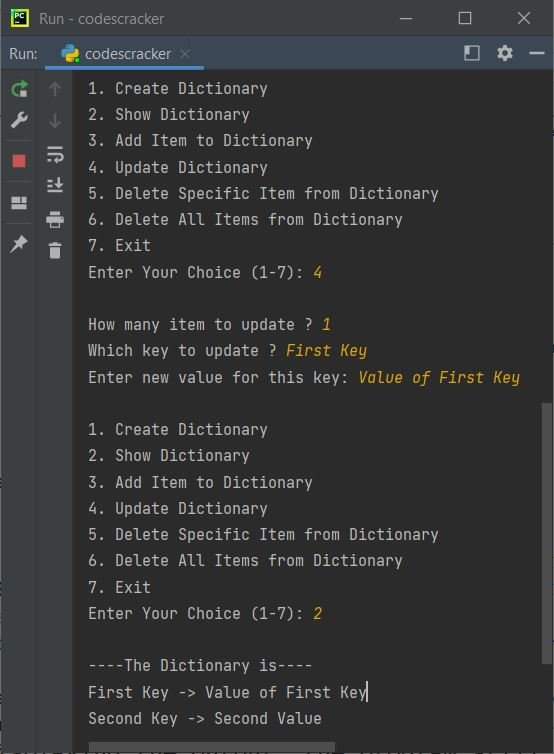The program is created in a way to handle all the errors when the user enters any invalid input. You can check it out for yourself. It will enhance your skills when you cross-check the program.

Python Online Test

« Previous Tutorial Next Tutorial »